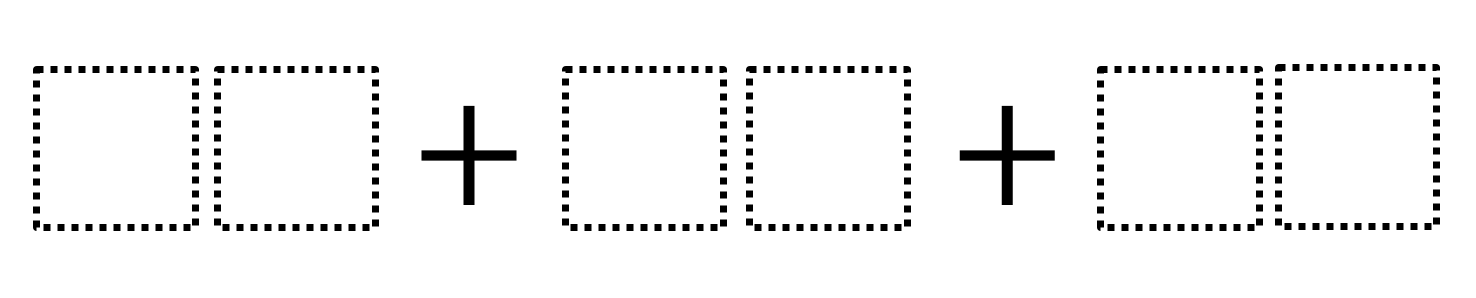Home > Grade 8 > Geometry > Interior Angles

# Interior Angles

Directions: Using the digits 0 through 9 at most one time, fill in the boxes to make the sum of the interior angles of a triangle.### Hint

What is the sum of the interior angle of a triangle?

Multiple answers. Possible answers: 67 + 89 + 24; 80 + 74 + 26

Source: Ashley Henderson

## Minimize Slope

Directions: Given the point (3,5), use digits 1-9, at most one time, to find a …

### One comment

1.Once given the hint, the problem doesn’t really do much for furthering students’ understanding of the triangle sum theorem, but my students had a great time making sense of the problem and then persevering to find as many answers as possible. Used in an intervention class and overheard a student say, “It’s been a while since I’ve had this much fun in math class” (since I made it a competition) and another student agree.